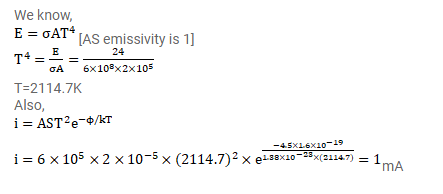# The constant A in the Richardson-Dushman equation for tungsten

Question:

The constant $\mathrm{A}$ in the Richardson-Dushman equation for tungsten $60 \times 10^{4} \frac{\mathrm{A}}{\mathrm{m}^{2}}-\mathrm{K}^{2}$.

The work function of tungsten is $4.5 \mathrm{eV}$. A tungsten cathode having a surface are $2.0 \times 10^{-5} \mathrm{~m}^{2}$ is heated by a $24 \mathrm{~W}$ electric heater. In steady state, the heat radiated by the cathode equals the energy input by the heater and the temperature becomes constant. Assuming that the cathode radiates like a blackbody, calculate the saturation current due to thermions. Take Stefan constant= $6 \times 10^{-8} \frac{\mathrm{W}}{\mathrm{m}^{2}}-\mathrm{K}^{4}$. Assume that the thermions take only a small fraction of the heat suplied.

Solution: